# Integer facts for kids

Kids Encyclopedia Facts

Integers are the natural numbers and their negatives.

These are some of the integers: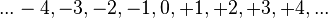${...-4, -3, -2, -1, 0, +1, +2, +3, +4,...}\,\!$

Zero is also an integer but it is not positive nor negative. "Integer" is another word for "whole". An integer is a rational number with no "fraction", or part. An integer is a decimal number with all zeros after the decimal separator. (For example, the integer 17 is the same as the decimal 17.0 or 17.0000.)

An integer has a next smaller number and a next larger number. There is no smallest integer. There is no largest integer. Each integer is either larger than, equal to, or smaller than any integer. Consecutive integers are integers that come after each other, like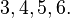${3, 4, 5, 6.}\,\!$

The sum of integers is an integer. The difference between integers is an integer. The product of integers is an integer. (For example, (12 + 2345 x (67 - 8) ) x 9 is an integer.) An integer divided by an integer is sometimes not an integer. (For example, 123 / 45 is not an integer.)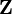$\mathbf{Z}$ or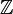$\mathbb{Z}$ is the name of the set of integers. ($\mathbf{Z}$, +, 0) is an abelian group.

The number of integers is infinite. The cardinal number of$\mathbf{Z}$ is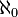$\aleph_0$. The ordinal number of$\mathbf{Z}$ is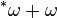$^*\omega + \omega$.

To sum up, an integer is a whole number that has no decimals.

## In programming

In some programming languages, like C, there are types called "int" or "integer." Integers can also be shown on a number lineInteger Facts for Kids. Kiddle Encyclopedia.